Worksheet Question: Data Handling

# Worksheet Question: Data Handling - Notes | Study Mathematics (Maths) Class 6 - Class 6

 1 Crore+ students have signed up on EduRev. Have you?

Q.1. Reena collected the data for the ages (in years) fo her hobby class group and she records the findings in the manner shown below :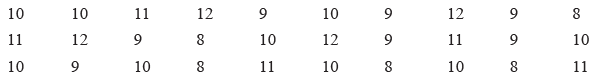Represent the above data using tally marks in the table below :

 Ages (in years) Tally Marks Frequency 8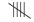05 9 10 11 12

(i) Find the age that appeared maximum number of times?
(ii) Find the ages that appeared equal number of times.
(iii) How many children are below 11 years?
(iv) How many children are of 10 years or above?

Ans.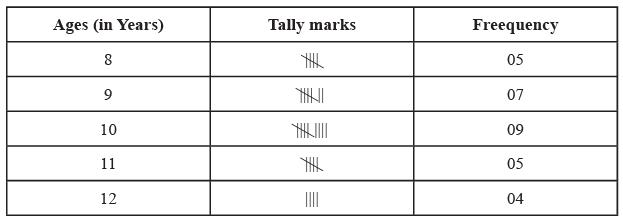(i) The age that appeared maximum number of times is 10 years.
(ii) 8 years & 11 years of age appeared equal number of times i.e. 5 times.
(iii) The total number of children below 11 years are 5 + 7 + 9 = 21
(iv) The total number of children that are of 10 years and above are 9 + 5 + 4 = 18

Q.2. The bar graph below is showing the liking of different fruits by students :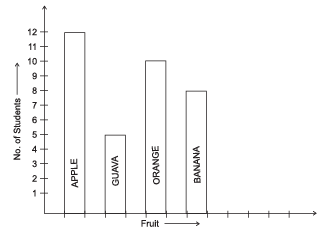(i) Which fruit is liked by most students?
(ii) How many students like orange?
(iii) How many more students like banana than guava?

Ans.
(i) The fruit is liked by most students is Apple.
(ii) 10 students like the fruit orange
(iii) Number of students who like banana = 8

Number of students who like guava = 5

Number of more students who like banana than guava = 8 -5 = 3

Q.3. Following is the production of cars in six months by a company.

 Month Production January 2000 February 1000 March 2400 April 800 May 1400 June 1800

Take 1 unit = 200 cars and draw a bar graph to represent the information.

Ans.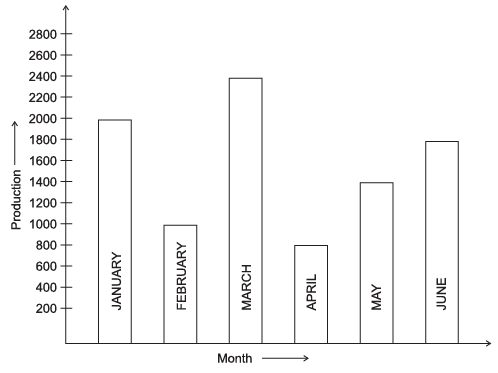Q.4. Following is the pictograph of class VI students. Here 1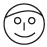= 10 boys and 1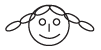= 10 girls. Looking at the pictograph below, answer the following questions.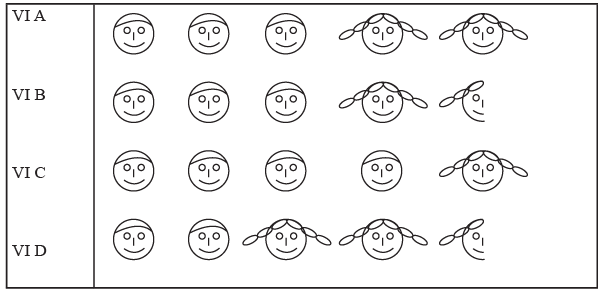(i) How many students are there in VI A, VI B, VI C and VI D sections each? __________
(ii) Which section has maximum number of boys? __________
(iii) Which section has maximum number of girls? __________
(iv) What is the total strength of class VI? __________

Ans.

(i) VI A =  50 students
VI B = 45 students
VI C = 50 students
VI D = 45 students
(ii) Section C has the maximum number of boys.
(iii) Section D has the maximum number of girls.
(iv) Total strength of class VI is 50 + 45 + 50 + 45 = 190 Students

Q.5. Number of students present in calss VI during a particular week are given below. It is represented by a pictograph where 1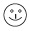= 6 students.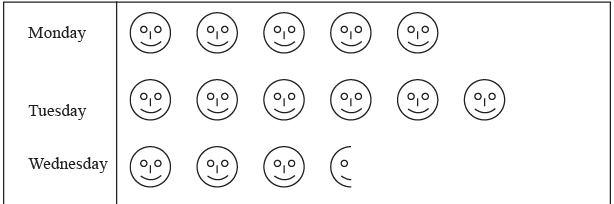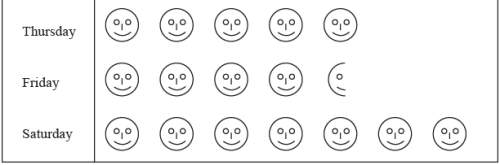(i) On which day maximum students were present? __________
(ii) On which day minimum students were present? __________
(iii) How many students were present on wednesday and Thursday each? __________

Ans.
(i) On Saturday maximum students were present.
(ii) On Wednesday minimum students were present.
(iii) 21 students were present on Wednesday and 30 students were present on Thursday.

Q.6. A survey showed the preference of different subjects by students of class VI

 Subject No. of Students English 25 Hindi 30 Maths 50 Science 35 Social Science 40

Draw a pictograph to illustrate the above data taking a scale of  1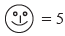students.

Ans.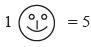students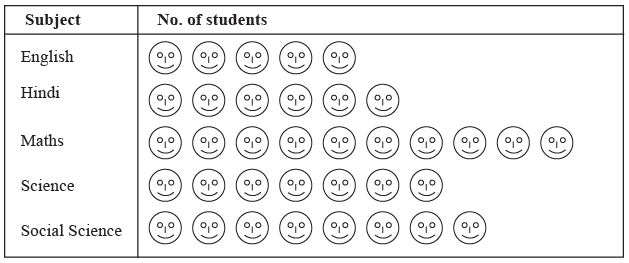The document Worksheet Question: Data Handling - Notes | Study Mathematics (Maths) Class 6 - Class 6 is a part of the Class 6 Course Mathematics (Maths) Class 6.
All you need of Class 6 at this link: Class 6

## Mathematics (Maths) Class 6

168 videos|282 docs|43 tests
 Use Code STAYHOME200 and get INR 200 additional OFF

## Mathematics (Maths) Class 6

168 videos|282 docs|43 tests

Track your progress, build streaks, highlight & save important lessons and more!

,

,

,

,

,

,

,

,

,

,

,

,

,

,

,

,

,

,

,

,

,

;